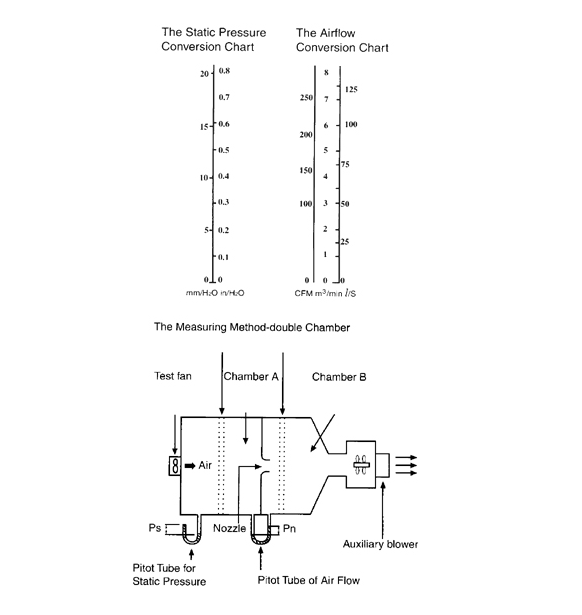# 风扇风量测试

Q = 60 A x V

Q: air flow rate (M3/min)
A: nozzle sectional area (M2)
V: average flow velocity through nozzle (M/sec)

V=[2 g Pn / r]

g: gravitational acceleration, 9.8m/s2
Pn: differential pressure (mm H2O)
Ps: static pressure
r: specific gravity of air, 1.2 kg/M3 at 20oC, 1atm